# 5.1速度

## 5.1.1向量与速度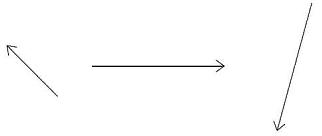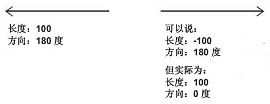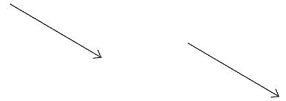## 5.1.2一个轴上的速度

package
{
import flash.display.Sprite;

public class Ball extends Sprite {
private var color:uint;

this.color=color;
init();
}

public function init():void {
graphics.beginFill(color);
graphics.endFill();
}
}
}

package
{
import flash.display.Sprite;
import flash.events.Event;

public class Velocity1 extends Sprite
{
private var ball:Ball;
private var vx:Number = 5;

public function Velocity1()
{
init();
}

private function init():void
{
ball = new Ball();
ball.x = 50;
ball.y = 100;
}

private function onEnterFrame(event:Event):void
{
ball.x +=  vx;
}
}
}

## 5.1.3两个轴上的速度

package
{
import flash.display.Sprite;
import flash.events.Event;

public class Velocity2 extends Sprite
{
private var ball:Ball;
private var vx:Number = 5;
private var vy:Number = 5;

public function Velocity2()
{
init();
}

private function init():void
{
ball = new Ball();
ball.x = 50;
ball.y = 100;
}
private function onEnterFrame(event:Event):void
{
ball.x +=  vx;
ball.y +=  vy;
}
}
}

## 5.1.4角速度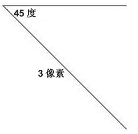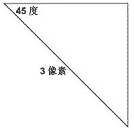vx = Math.cos(angle) * speed;
vy = Math.sin(angle) * speed; 

package
{
import flash.display.Sprite;
import flash.events.Event;

public class VelocityAngle extends Sprite
{
private var ball:Ball;
private var angle:Number = 45;
private var speed:Number = 3;

public function VelocityAngle()
{
init();
}

private function init():void
{
ball = new Ball();
ball.x = 50;
ball.y = 100;
}
private function onEnterFrame(event:Event):void
{
var radians:Number = angle * Math.PI / 2;
var vx:Number = Math.cos(angle) * speed;
var vy:Number = Math.sin(angle) * speed;
ball.x += vx;
ball.y += vy;
}
}
}

### 向量加法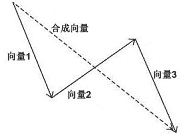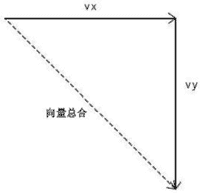### 鼠标跟随

package
{
import flash.display.Sprite;
import flash.events.Event;

public class FollowMouse extends Sprite
{
private var arrow:Arrow;
private var speed:Number = 5;

public function FollowMouse()
{
init();
}

private function init():void
{
arrow = new Arrow();
}
private function onEnterFrame(event:Event):void
{
var dx:Number = mouseX - arrow.x;
var dy:Number = mouseY - arrow.y;
var angle:Number = Math.atan2(dy, dx);
arrow.rotation = angle * 180 / Math.PI;
var vx:Number = Math.cos(angle) * speed;
var vy:Number = Math.sin(angle) * speed;
arrow.x += vx;
arrow.y += vy;
}
}
}

## 5.1.5速度扩展

Sprite，影片剪辑或任何的显示对象都有许多属性可以使用，而这些属性大多数都有比较大的取值范围，可以让我们制作出多种多样的动画。也许，速度一词用于这些属性上并不合适，但是在概念上是相同的，所以我也通常使用 v(velocity) 为变量命名的首字母。

package
{
import flash.display.Sprite;
import flash.events.Event;

public class RotationalVelocity extends Sprite
{
private var arrow:Arrow;
private var vr:Number = 5;

public function RotationalVelocity()
{
init();
}

private function init():void
{
arrow = new Arrow();
arrow.x = stage.stageWidth / 2;
arrow.y = stage.stageHeight / 2;
}
private function onEnterFrame(event:Event):void
{
arrow.rotation += vr;
}
}
}

arrow.x += vx;
arrow.y += vy;
arrow.alpha += vAlpha;
arrow.rotation += vr;
arrow.scaleX = arrow.scaleY += vScale;
// etc. 

2006 - 2023，推荐分辨率1024*768以上，推荐浏览器Chrome、Edge等现代浏览器，截止2021年12月5日的访问次数：1872万9823 站长邮箱